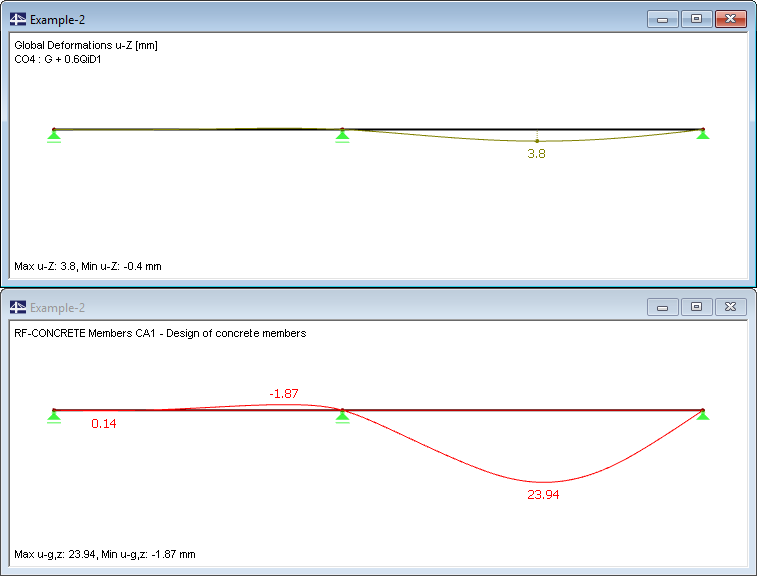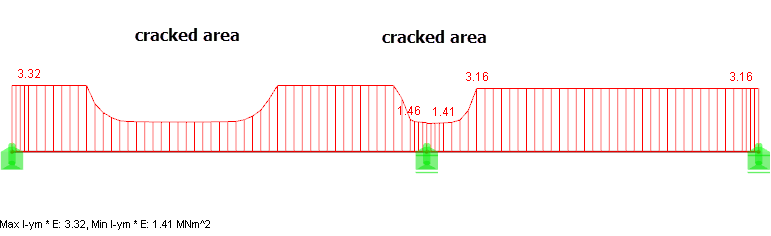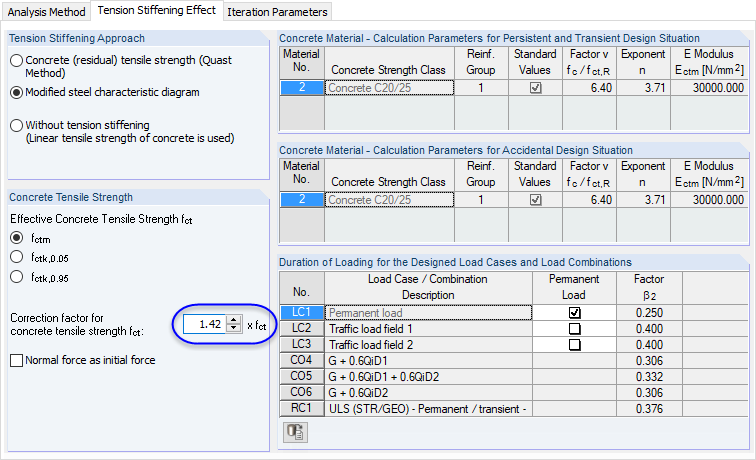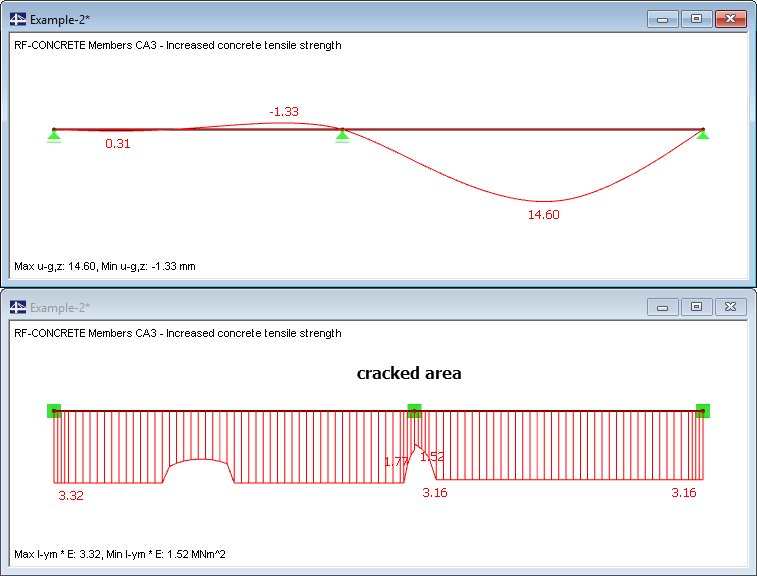# RF-CONCRETE Members – Online Manual Version 5

Online manuals, introductory examples, tutorials, and other documentation.

# 9.2.7 Results Evaluation

### Results EvaluationFigure 9.28 Deformations according to linear elastic calculation (above) and nonlinear calculation with creep (below)

The deformation from the nonlinear calculation with consideration of the creep effect proves to be significantly higher than the deformation from the pure linear elastic calculation without creep effect. As described in chapter 9.2.5, the calculated deformation falls below the recommended limit value of ℓ/250.

The deformations in state II are considerably affected by three factors:

Floor thickness

In our example, the floor thickness was determined by a limitation of the bending slenderness according to DIN 1045-1, 11.3.2, so that we could describe the calculation process. According to EN 1992-1-1, the result is a floor thickness of h ≥ 18 cm calculated with the same boundary conditions. By increasing the thickness to 18 cm we can significantly reduce the deformations.

Creeping

The assumed creep coefficient appears to be relatively high at φ = 2.95 but meets the requirements according to EN 1992-1-1 for the assumed environmental conditions and the cross-section geometry.

By means of the factor ψ (ψ2,1 = 0.6) used to calculate the quasi-permanent action combination, it would be possible to effect a certain reduction from creep-producing load to acting load.

Concrete tensile strength

The distribution of stiffnesses in the figure below shows that a large area in span 1 is cracked in the serviceability limit state.Figure 9.29 Stiffness diagram Iy,m ⋅ E over beam length
Alternative calculation with increased concrete tensile strength

For the calculation, the concrete tensile strength was assumed with the value fctm (axial tensile strength) according to EN 1992-1-1. Parameters such as the gradient of stresses have a great influence on the concrete's effective tensile strength: A large stress gradient increases the tensile strength because the corresponding high stresses act only in few fibres. You can find more information about the different influencing factors that affect the tensile strength, inter alia, in .

For our example, the tensile strength is calculated once more according to , chapter 2.1.1:

• fctm = 0.45 ⋅ 0.818 ⋅ 1 ⋅ 25 2/3 = 3.14 N/mm2

where

 fcm = 20 + 5 = 25 N/mm2 The mean value is taken into account by the summand 5 N/mm2 CV = 0.85 - 0.2 ⋅ 0.16 = 0.818 ≥ 0.65 Prior damage of structural component is taken into account Ch = (2.6 + 24 ⋅ 0.16)/(1.0 + 40 ⋅ 0.16) = 0.87 Influence of structural component thickness Cη = 1 Influence of eccentricityη = M/(N ⋅ h) → ∞ für N → 0To consider the influence of an increased tensile strength, the model is calculated in a second design case using the adjustment factor 3.14/2.2 = 1.42 (see Figure 9.30).Figure 9.30 Adjusting the concrete tensile strength in the Settings for Nonlinear Calculation dialog box

The calculation shows a strong reduction of the cracked zones that also leads to a reduction of the deformation to ul = 14.6 mm. This value clearly lies below the reference value of ℓ /250 = 5,000/250 = 20 mm.

The following figure illustrates the relation between deformation and stiffness reduction. In span 1, the start of the crack formation is discernible; only in the support area does the cross-section pass locally into the cracked state.Figure 9.31 Deformation and bending stiffness for increased concrete tensile strength (stress gradient)

It becomes evident how sensitively the nonlinear calculation reacts to changed parameters. The difference is especially significant for structural components with large stiffness changes between cracked and uncracked state.

Literatur
  Noakowski, Piotr u. Schäfer, Horst. Steifigkeitsorientierte Statik im Stahlbetonbau. Ernst & Sohn Verlag, 2003.